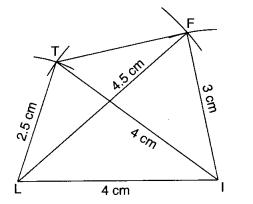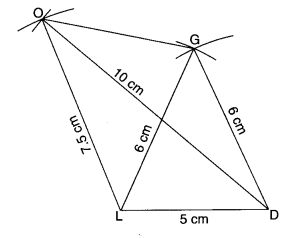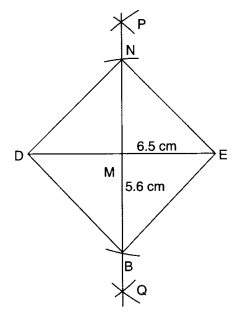# NCERT Solutions for Class 8 Maths Chapter 4 Practical Geometry Ex 4.2

NCERT Solutions for Class 8 Maths Chapter 4 Practical Geometry Ex 4.2 are part of NCERT Solutions for Class 8 Maths. Here we have given NCERT Solutions for Class 8 Maths Chapter 4 Practical Geometry Ex 4.2.

 Board CBSE Textbook NCERT Class Class 8 Subject Maths Chapter Chapter 4 Chapter Name Practical Geometry Exercise Ex 4.2 Number of Questions Solved 1 Category NCERT Solutions

## NCERT Solutions for Class 8 Maths Chapter 4 Practical Geometry Ex 4.2

Question 1.
LI = 4 cm
IF = 3 cm
TL = 2.5 cm
LF = 4.5 cm
IT = 4 cm

OL = 7.5 cm
GL = 6 cm
GD = 6 cm
LD = 5 cm
OD = 10 cm

(iii) Rhombus BEND
BN – 5.6 cm
DE = 6.5 cm
Solution.
(i) Steps of Construction

1. Draw LI = 4 cm.
2. With L as center and radius LT = 2.5 cm, draw an arc.3. With I as center and radius, IT = 4 cm, draw another arc to intersect the arc drawn in step 2 at T.
4. With I as center and radius IF = 3 cm, draw an arc.
5. With L as center and radius LF = 4.5 cm, draw another arc to intersect the arc drawn in step 4 at F.
6. Join IF, FT, TL, LF and IT.

Then, LIFT is the required quadrilateral.

(ii) Steps of Construction

1. Draw LD = 5 cm.
2. With L as center and radius LG = 6 cm, draw an arc.3. With D as center and radius DG = 6 cm, draw another arc to intersect the arc drawn in step 2 at G.
4. With L as center and radius LO = 7.5 cm, draw an arc.
5. With D as center and radius DO = 10 cm, draw another arc to intersect the arc drawn in step 4 at O.
6. Join DG, GO, OL, LG and DO.

Then GOLD is the required quadrilateral.

(iii) Steps of Construction

1. Draw DE = 6.5 cm.
2. Draw perpendicular bisector PQ of DE so as to intersect DE at M. Then M is the mid-point of DE.
3. With M as centre and radius
$$=\frac { 1 }{ 2 } \times \left( 5.6 \right) =2.8 cm$$
opposite sides of DE to intersect MP at N and MQ at B.4. Join DN, NE, EB, and BD.

Then, BEND is the required rhombus.

We hope the NCERT Solutions for Class 8 Maths Chapter 4 Practical Geometry Ex 4.2 help you. If you have any query regarding NCERT Solutions for Class 8 Maths Chapter 4 Practical Geometry Ex 4.2, drop a comment below and we will get back to you at the earliest.